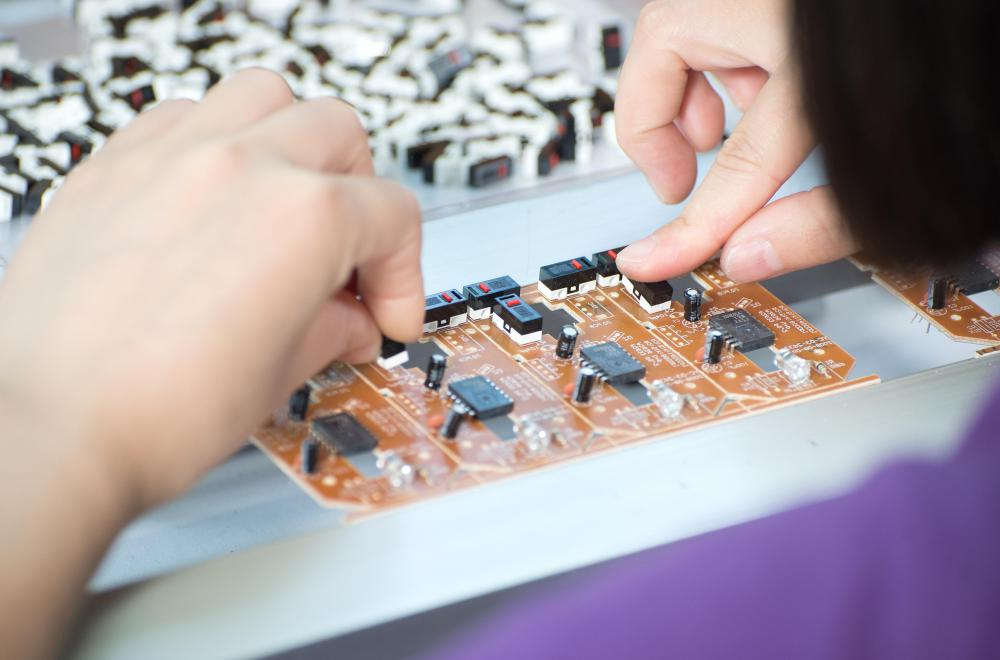# What Is a Mesh Analysis?

Helen Akers

A mesh analysis is a set of mathematical equations that help determine the paths of electrical currents. The analysis process uses Ohm's law and Krichhoff's voltage law to examine how different points on a circuit board form communication bonds. It involves isolating a circuit board's loops, identifying opposite voltage charges, and substituting values into equations to solve the unknown direction of currents. The mesh analysis is one of many methods used to analyze electrical currents and is also known as the loop current method.Part of a mesh analysis shows what loops exist between different circuits on a single board or in an entire interdependent set of circuits.

Loops are one way to tell if a circuit board or network that relies on circuits is operational and working properly. Part of a mesh analysis shows what loops exist between different circuits on a single board or in an entire interdependent set of circuits, such as a telecommunications network. Loops can be thought of as relationships between separate critical points or nodes. The electrical current sent between points or nodes makes the communication process and transfer of information possible.

Once a circuit board's loops are determined, the next step in a mesh analysis is to identify each node's electrical charge. One of the characteristics of a loop is that it occurs between two nodes that have opposite voltage charges. For example, one of the nodes on a board that is labeled "A" may have a negative charge, while a node labeled "B" may have a positive charge. During the analysis process, the voltage charges are assumed according to the proposed flow of the current.

The most complicated part of the mesh analysis process is forming the equations that reveal whether the assumed current direction is correct or incorrect. Krischhoff's voltage law takes the first circuit's resistor's voltage value and adds it to the amount of the unknown value of the first current and the second current, which is then multiplied by two. This result is also added to the unknown value of the first current multiplied by four. The value of the first side of the equation is set to equal zero and is solved algebraically.

By isolating the unknown values of both circuits, the values for the first and second circuits reveal which side is truly negative and positive. The mesh analysis reveals the correct direction of the electrical current by showing which side accepts the downward and upward flows. Negative charges that flow through a resistor indicate a upward flow, while positive charges reflect a downward path.

## You might also Like